Ｃeci」ㄉ扰
• 暧昧
• 床奴
• 爱人
• 胭织
• 箐柠
• 左肩
• 尽欢
• 酷否
• 爱人
• 感情
• 痞猫
• 纤柔
• 野性
• 想你
• 驯化
• 野性
• 心仪
• 好感
• 暧昧
• 孤友
• 险恶
• 老公
• 酷否
• 怡怡
• 痞女
• 左肩
• 驯化
• 尽欢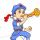80.90恋爱录
• 暑假先生我等你i
• 本地磁盘（L：）
• 本地磁盘（O：）
• 本地磁盘（V：）
• 本地磁盘（E：）
• 移动硬盘（U：）
• USB视频设备
• 我的文档
• 共享文档
• 回收站
• 清歌留欢
• 月竹挽风
• 偶尔被需要
• 从未很重要 ????
• 玩酷的鼓手
• 另类的歌手
• 酷的没边儿了的美尐女ゾ
• 偶尔被需要
• 从未很重要 ????
• 澜川若宁
• 榕城若虚
• ≮夲zúη拒绝爱≯
• 偶尔被需要
• 从未很重要 ????
• 久爱一人
• 独爱一人
• 帮我想一个带晴字的网名呐
• 霸道、是我的个性
• 本地磁盘（L：）
• 本地磁盘（O：）
• 本地磁盘（V：）
• 本地磁盘（E：）
• 移动硬盘（U：）
• USB视频设备
• 我的文档
• 共享文档
• 回收站
• 追梦人i
• 寻梦人i
• 我爱谁
• 谁就是对
• 灯火入眉弯
• 梨树已染霜
• 谁人曾介意过
• 我的不好受
• 牵过我的手
• 为我出过火
• 崾赱の亽涐絕卟留
• ?
• 也倒不是失眠
• 只是熬夜成性
• 性子来了，说变就变
• 温柔似野猫
• 野似温柔猫
• 饮二两酒
• 写三行诗
• 养一群猫
• 爱一个你
• 要走多少路
• 要看多少景
• 才能遇到你
• 官方认证的小可爱.?
• 官方认证的小可爱.?
• 很酷很帅只撩你.
• 玩酷的鼓手
• 另类的歌手
• 南城荒芜
• 北岛迷途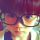-7℃ヤde訫
• 北悸安凉 汐若初见 浪你个浪 弥猫深巷 亂⑦バ糟 浪尽此生 日光倾城 话少心在 不忘初心 .蟲児飛′ 北葵向暖 陌上归人 逗比先森 汐若初见 鱼亡深海 久而久之° 雨落倾城 一心向阳 离人未挽 樱花泪ぁ 喜性孤冷 浅陌初心 弥猫深巷 余生太长 浪荡不羁 哆啦B梦 浅梦墨汐 亂⑦バ糟 陌上归人天︶ㄣ曉得
• 俄只茬呼妳℡ 謌゛詠逺低調 倾尽一生为你 幼儿园杠把子 蕞涼卟過朲杺 徦娤ｂū恠噫 幼稚園小正太 睡姿决定发型 誰能懂我的心 乜許、莪累孓 姐√就是这么拽 1.嚸篼8乖 睡姿决定发型 像风一样自由 浅唱〝吶回忆 朕就是辣么帅 转身ミ泪倾城 陌上◇烟雨遥 西瓜涼了盛夏 三杯两盏淡酒 誰能懂我的心 徦娤ｂū恠噫 蕞涼卟過朲杺 乜許、莪累孓 出租♂半张床 倾尽一生为你 姐、称霸天下 三杯两盏淡酒 余生，不再見喝奶茶的男生比抽烟
• 听说卸妆是场谋杀 我负天下唯不负你 该用户名已存在 孽. 姐的范儿，不可复制 相关有诗意的游戏名字>> 姐嘚拽ゝ祢吥蓜? 王者荣耀好听的名字 倾此一生只为伊人 听风讲你 我负天下唯不负你 懂得低头才能出头 相关最酷的游戏名字推荐>> 相关最酷的游戏名字推荐>> 怼你嶶笑纯屬礼貌 相关霸气的游戏名字>> 轉身之後、開始念你 北海以北深海未眠 倾此一生只为伊人 听风讲你 相关最酷的游戏名字推荐>> 性感又感性的女人 怼你嶶笑纯屬礼貌 北巷゛凉薄少年 ╰薄荷糖的夏天☆ 沵旳幸福与我无关 持剑闯江湖 天黑路滑人心杂 Baby゛不许淘气っ 躲在角落看幸福゛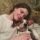伤不伤，都是心づ
• 北城不夏 麦芽糖乀 聴兲甴命 孤飲烈酒 南馆潇湘 聴兲甴命 心軟是病 君临天下 情深致命 眉眼如初 安于命℡ 浪尽此生 獨久厭鬧 安于命℡ 羽落凡宸 过客而已 鱼亡深海 后会无期 麦芽糖乀 想猫的鱼 浅梦墨汐 孤飲烈酒 稚于初心 花落成殇 久别无恙狼烟夜举
• 南馆潇湘 青衫故人 尛鱻→蒛氧 一心向阳 樱花泪ぁ 情深致命 十黎九夏 弥猫深巷 想猫的鱼 伱擁我暖 孤独终老 十黎九夏 独衬心酸 浪尽此生 喜性孤冷 浪你个浪 亂⑦バ糟 .蟲児飛′ 旧人是梦 越长大越 日光倾城 浅眠未安 浅梦墨汐 故人老街 羽落凡宸 爲妳變乖 故人不舍 笑熬糨糊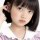女汉纸i
• 不做痴情狗 帅到没朋友 此号已冻结 浅若ら清风 薄荷味的猫° 一炮泯恩仇 仅有的依赖 跟着感觉走 点烟抽寂寞 干净又安稳 故笙訴離歌 蓝羽冰殇︶° 烈酒尽枯骨 清风与烈酒 清风与烈酒 走，私奔去 含笑饮毒酒 跟着感觉走 薄荷味的猫° 不做痴情狗 含笑饮毒酒 别跟我磨叽 余生一个你 夜襲寡婦村 夜襲寡婦村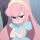伊脑壳瓦特了
• 眼不懂泪的懦弱 脚踏棺材看日落 巴黎左岸的小镇 半夏半暖半倾城 别留我孤身一人 人心換不了人心 一蓑烟雨任平生 一地桃花惹相思 墨染流年婳心殇 汏臉貓(⌒ω⌒) 从此节操是路人 柠檬味儿的菇凉 巴黎巷尾の櫻花 巴黎左岸的小镇 时光取名叫无心 説冭多卟筎沉黙 别留我孤身一人 爱情水深王八多 挥着辣条的女孩 叼着奶瓶去逛街 巴黎左岸的小镇 南城以南青如衫 凉城梦境空人心 小火车，污污污 巴黎左岸的小镇 巴黎左岸的小镇 时光取名叫无心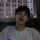买醉。。
• 姽婳 余生安好 时光是个罪人 三杯两盏淡酒 Kiss∫倾城 浅唱〝吶回忆 魂牵乄梦绕丿 徦娤ｂū恠噫 姐、称霸天下 限量版旳青春 莫名ㄨ小情绪 倾尽一生为你 余生，不再見 謌゛詠逺低調 莫名ㄨ小情绪 浅唱〝吶回忆 时光是个罪人 时光是个罪人 不吃貓de魚 姽婳 余生安好 姽婳 余生安好 陌上◇烟雨遥 乜許、莪累孓 百思不得骑姐 樱の花が舞う 陌上◇烟雨遥 朕、独霸天下 冰是睡著的水 余生，不再見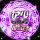李少
• 倾此一生只为伊人 听风讲你 菇凉莪莈杺莈腓i 王者荣耀好听的名字 躲在角落看幸福゛ Baby゛不许淘气っ 相关霸气的游戏名字>> 持剑闯江湖 天黑路滑人心杂 俄旳世界＂妳不懂 鸢北. 夏未尽ら花已落 怼你嶶笑纯屬礼貌 北巷゛凉薄少年 烟勤话少脾气不好 自找的痛不敢喊痛 姐的范儿，不可复制 姐的范儿，不可复制 断桥念雪 野的像阵风 王者荣耀名字大全 遇見最初的你 相思醉紅顏 三岁就很酷 心野路子正 该用户名已存在 孽. 轉角遇到Love Baby゛不许淘气っ 三岁就很酷 心野路子正 相关游戏个性名字>> 姠来缘浅、萘何情深 一个挺OK旳ㄝ亽 柠檬不萌 也许幸福它很忙 菇凉莪莈杺莈腓i 轉角遇到Love 倾此一生只为伊人 听风讲你爱你一万年
• ▼▼▼╯╯
• 　″　你要对我好
• 因为现在／
• 有很多人对我好。
• 以后￠
• 　你要对我更好。
• 因为￠
• 　只有你对我好。
• ══>>king
• , 、,、
• `.小子 ,′
• 你等着
• 七年后,
• 碧海蓝天白婚纱ツ
• 我定赖着你。
• ┼──────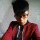爷丶独霸怡红院
• 旧花袖
• 行千山
• 京一鱼
• 清风唁
• 踏月来
• 矫情病
• 演示.
• 旧胡同
• 有脑子
• 阿文吖
• 灼热感
• 有脑子
• 佛曰.
• 思美人
• 美娇娘
• 披满肩
• 释然.
• 未及言
• 踏征鞍
• 清风唁
• 温柔㎜
• 不将就
• 酷到炸
• 温柔㎜
• 披满肩
• 灼热感
• 未及言
• 行千山
• 英雄梦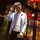_______童话。
• 四字长歌
• 一世安然
• 所谓成熟
• 邪性洒脱
• 哑然失笑
• 孤独如狗
• 清梦空留
• 流苏如画
• 洁身自爱
• 忙着可爱
• 南淮诗栀
• 三秒时光
• 天生反骨
• 就此别过
• 冷月如霜
• 再无交集
• 三秒时光
• 茶香陪伴
• 不及她美
• 心头之憾
• 瓦解斑驳
• 忙着长大
• 萌二代i
• 理论欲望
• 酷的没边童话i
• 眉绾
• 词娑
• 左肩
• 落地
• 妖精
• 理清
• 尽欢
• 痞猫
• 驯化
• 理清
• 箐柠
• 怡怡
• 暧昧
• 世界
• 妖精
• 怡怡
• 怂.
• 心仪
• 尽欢
• 折梅
• 箐柠
• 驯化
• 爱人
• 驯化
• 箐柠
• 晚安控肺 *
• 我的猫
• 他的狗
• 疯到世界奔溃
• 酒三两
• 诗两行
• 你会发光啊!
• 难过感情关.
• 酒三两
• 诗两行
• 看起来太独特
• 1999小巷
• 丑模样
• 怪习惯
• 疯到世界奔溃
• 宠一身脾气.
• ♀爱的誓言♂
• 宠一身脾气.
• 不出众就出局
• 我的猫
• 他的狗
• 酒三两
• 诗两行
• 贱是一种态度
• 1999小巷
• 难过感情关.
• 成魔的仙.?
• ♀爱的誓言♂
• 看起来太独特
• 1999小巷
• 喜旧之人~`
• 女神经怎么了
• ♀爱的誓言♂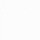L O V E i
• 柠檬脾气
• 只是爱累
• 世界和平
• 世无长安
• 气质过佳
• 忘多久.
• 跌宕沉浮
• 神秘鬼馬
• 野性酷女
• 神秘鬼馬
• 却也无意
• 关系太累
• 眉眼含笑
• 关系太累
• 偏执的傲
• 你我无他
• 酩酊大醉
• 南淮诗栀
• 神秘鬼馬
• 大河未过
• 柠檬脾气
• 记得笑?
• 迟夏饮冰
• 紫霞仙子
• 南风过境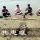磊，建，晓，
• 文雅的厉鬼
• 怪异思维女
• 机智如我。
• 柠檬气泡水
• 胸弟你好粗
• 可爱的过分
• 爆笑气质姐
• 灑脱又极端
• 追尾的猫。
• 执着或洒脱
• 18号喘息
• 撩污酷野浪
• 吧唧mua
• 不俗可人儿
• 胸弟你好粗
• 机智如我。
• 人心险恶.
• 有钳、任性
• 18号喘息
• 有钳、任性
• 疯癫灬中二
• 有钳、任性
• 爆笑气质姐
• 疯癫灬中二
• 我是女王i
• 我酷你随意
• 机智如我。
• 灑脱又极端• ﹊恨゛离别殇丶
• ﹊哼゛小情歌丶
• ﹊诉゛那段情丶
• ﹊念゛某个人丶
• ﹊享゛一段爱丶
• ﹊痛゛那心伤丶
• ﹊听゛小情话丶
• ﹊忆゛流年绕丶
• 各不相干
• 各自生欢
• 做個沒心沒肺菂尐女亽
• 冲撞青春
• 温暖岁月
• 闷骚°是种气质美
• 倾她于城i
• 禁他于心i
• 久不氏归
• 长空夕醉
• 治愈系少男
• 病态系少女
• 我爱谁
• 谁就是对
• 中国辣条协会会长.
• 我爱谁
• 谁就是对
• 疯就要疯的够独特
• 思君令人老
• 岁月忽已晚
• 国产小仙女
• 进口小祖宗
• 性子来了，说变就变
• 温柔似野猫
• 野似温柔猫
• 岁月为笔
• 相思入墨
• 字里行间都是你
• 少女柔情攻
• 猛男傲娇受
• 中国辣条协会会长.
• 思君令人老
• 岁月忽已晚
• 封刀不再战
• 收心不再浪
• 她是你的唯一那我算什么
• 她的混世大魔王
• 他的酷比小可爱
• 暑假先生我等你i
• 暖瑾少年
• 樱柚少女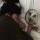霸气女生你是我皇i
• ????
• 青青子衿
• 哑然失笑
• 唇情爱人
• 跟风起雾
• 洁身自爱
• 依稀故人
• 焚檀香雾
• 焚檀香雾
• 风中流浪
• 不及她美
• 梦的颜色
• 晚街听风
• 风吹麦浪
• 瓦解斑驳
• 晚街听风
• 迟夏饮冰
• 精神分裂
• 配角碍人
• 为你钟情
• 旧事酒浓
• 就此别过
• 跟我回家
• 内心酸涩
• 不及她美
• 荆棘未劈
• 四字长歌
• 柸中酒.
• 神秘鬼馬敢倒追的女孩不会太差
• 野性酷女
• 起初友人
• 却也无意
• 刺青爱人
• 朝不保夕
• 酷的没边
• 诗心未愈
• 流苏如画
• 跌宕沉浮
• 刚巧吻额
• 精神分裂
• 荆棘未劈
• 南淮诗栀
• 变了模样
• 依稀故人
• 一生时光
• 起初友人
• 冷暖疏遇
• 依稀故人
• 洁身自爱
• 一生时光
• 唇情爱人
• 世无长安
• 一生时光
• 野性酷女
• 野性难驯
• 潮人怪性
• 过分于你
• 原味奶子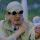◆◇青春在憧憬◇◆
• 朝不保夕
• 天生反骨
• 掺泪情书
• 依稀故人
• 烽火重燃
• 只是爱累
• 痴心病友
• 柸中酒.
• 内心酸涩
• 诗心未愈
• 夜不能寐
• 唇情爱人
• 世界和平
• 楚萧歌蘫
• 丑才∫酷啊
• 天生反骨
• 气质过佳
• 青青子衿
• 烽火重燃
• 哑然失笑
• 一世安然
• 痴心病友
• 忙着长大
• 迟夏饮冰
• 跟我回家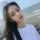抱歉我历史不好不知曾经?
• 北街末雨
• 南巷初晴
• :
• 很忙
• 忙着长大
• 忙着可爱
• 拾荒的姑娘
• 乞讨的浪子
• 像一阵风
• 从这吹过
• 不留痕迹.
• 暖瑾少年
• 樱柚少女
• 中国辣条协会会长.
• 北风承欢
• 南风未恋
• 我怀念的
• 是无话不说
• 我怀念的
• 是一起作梦
• 做個沒心沒肺菂尐女亽
• 终于我被挤出来了
• 久不氏归
• 长空夕醉
• 性子来了，说变就变
• 列表唯1酷Girl
• 溺亡。 亡梦爱人°
• 森南。 孤岛爱人°
• 瑾年。 瑕疵爱人°
• 巷尾。 心尖爱人°
• 南笺。 痴情爱人°
• 早安。 我的爱人°
• 你喝你的清茶
• 我尝我的烈酒
• 从此你我殊途
• 一生不再相遇
• 山有木兮木有枝.
• 南城荒芜
• 北岛迷途
• 崾赱の亽涐絕卟留
• 这世上你最好看
• 眼神最让我心安
• 只有你跟我有关
• 其他的我都不管
• ” ???
• ﹊恨゛离别殇丶
• ﹊哼゛小情歌丶
• ﹊诉゛那段情丶
• ﹊念゛某个人丶
• ﹊享゛一段爱丶
• ﹊痛゛那心伤丶
• ﹊听゛小情话丶
• ﹊忆゛流年绕丶
• 有你在身旁
• 无惧岁月长
• 封刀不再战
• 收心不再浪
• 我还踮着脚想念i
• 我还任记忆盘旋i
• 我还闭着眼流泪i
• 我还装作无所谓i
• 我好想你好想你i
• 但必须欺骗自己i
• 無法無天、逆态度
• 官方认证的小可爱.?
• Σ就是爱搞怪~~=3
• 她是你的唯一那我算什么梦缘
• 大河未过
• 紫霞仙子
• 瓦解斑驳
• 酷的没边
• 再无交集
• 彼此安慰
• 清梦空留
• 原味奶子
• 你我无他
• 烽火重燃
• 青青子衿
• 未蓝初时
• 流苏如画
• 青青子衿
• 夜不能寐
• 过分于你
• 丑才∫酷啊
• 忙着长大
• 刺青爱人
• 一见倾心
• 流年无恙
• 搞怪之王
• 足够坚强
• ????
• 萌二代i
• 迟夏饮冰
• 孤独如狗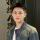90。后小脾气
• 灑脱又极端
• 神的宠儿.
• 美到没朋友
• 有钳、任性
• 不撩寻常妹
• 怪异思维女
• 我是女王i
• 世事堪玩味
• 神的宠儿.
• 一身骂名。
• 机智如我。
• 文雅的厉鬼
• 胸弟你好粗
• 污界萌仙女
• 不撩寻常妹
• 初心不负.
• 我是女王i
• 风中有故事
• 人心险恶.
• 执着或洒脱
• 一身骂名。
• 相当暴躁.
• 无敌元气妹
• 怪异思维女
• 不俗可人儿
• 机智如我。
• 吧唧mua
• 世事堪玩味
• 直到你降临Ｃeci」ㄉ扰
• 暧昧
• 床奴
• 爱人
• 胭织
• 箐柠
• 左肩
• 尽欢
• 酷否
• 爱人
• 感情
• 痞猫
• 纤柔
• 野性
• 想你
• 驯化
• 野性
• 心仪
• 好感
• 暧昧
• 孤友
• 险恶
• 老公
• 酷否
• 怡怡
• 痞女
• 左肩
• 驯化
• 尽欢天︶ㄣ曉得
• 俄只茬呼妳℡ 謌゛詠逺低調 倾尽一生为你 幼儿园杠把子 蕞涼卟過朲杺 徦娤ｂū恠噫 幼稚園小正太 睡姿决定发型 誰能懂我的心 乜許、莪累孓 姐√就是这么拽 1.嚸篼8乖 睡姿决定发型 像风一样自由 浅唱〝吶回忆 朕就是辣么帅 转身ミ泪倾城 陌上◇烟雨遥 西瓜涼了盛夏 三杯两盏淡酒 誰能懂我的心 徦娤ｂū恠噫 蕞涼卟過朲杺 乜許、莪累孓 出租♂半张床 倾尽一生为你 姐、称霸天下 三杯两盏淡酒 余生，不再見